Second Law Of Thermodynamics MCQ Level – 2 (Part - 2)

# Second Law Of Thermodynamics MCQ Level – 2 (Part - 2)

Test Description

## 10 Questions MCQ Test Topic wise Tests for IIT JAM Physics | Second Law Of Thermodynamics MCQ Level – 2 (Part - 2)

Second Law Of Thermodynamics MCQ Level – 2 (Part - 2) for Physics 2023 is part of Topic wise Tests for IIT JAM Physics preparation. The Second Law Of Thermodynamics MCQ Level – 2 (Part - 2) questions and answers have been prepared according to the Physics exam syllabus.The Second Law Of Thermodynamics MCQ Level – 2 (Part - 2) MCQs are made for Physics 2023 Exam. Find important definitions, questions, notes, meanings, examples, exercises, MCQs and online tests for Second Law Of Thermodynamics MCQ Level – 2 (Part - 2) below.
Solutions of Second Law Of Thermodynamics MCQ Level – 2 (Part - 2) questions in English are available as part of our Topic wise Tests for IIT JAM Physics for Physics & Second Law Of Thermodynamics MCQ Level – 2 (Part - 2) solutions in Hindi for Topic wise Tests for IIT JAM Physics course. Download more important topics, notes, lectures and mock test series for Physics Exam by signing up for free. Attempt Second Law Of Thermodynamics MCQ Level – 2 (Part - 2) | 10 questions in 45 minutes | Mock test for Physics preparation | Free important questions MCQ to study Topic wise Tests for IIT JAM Physics for Physics Exam | Download free PDF with solutions
 1 Crore+ students have signed up on EduRev. Have you?
Second Law Of Thermodynamics MCQ Level – 2 (Part - 2) - Question 1

### Consider a system of N atoms of an ideal gas of type A at temperature T and volume V. It is kept in diffusive contact with another system of N atoms of another ideal gas of type B at the same temperature T and volume V. Once the combined system reaches equilibrium. Select one:

Detailed Solution for Second Law Of Thermodynamics MCQ Level – 2 (Part - 2) - Question 1

The correct answer is: entropy of final system is equal to the sum of individual entropies

Second Law Of Thermodynamics MCQ Level – 2 (Part - 2) - Question 2

### A heat pump uses 300 J of work to remove 400 J of heat from the low-temperature reservoir. How much heat is delivered to a higher temperature reservoir?

Detailed Solution for Second Law Of Thermodynamics MCQ Level – 2 (Part - 2) - Question 2

W = 300 J

Q= 400 J

QH = W + QC

QH = 300 J + 400 J

QH = 700 J

Heat delivered to the higher temperature reservoir is 700 J.

Second Law Of Thermodynamics MCQ Level – 2 (Part - 2) - Question 3

### For the thermodynamics process shown in the figure, corresponding T-S diagram is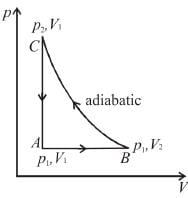Select one:

Second Law Of Thermodynamics MCQ Level – 2 (Part - 2) - Question 4

The T–S diagram of two cycles for the operation of an engine are shown in figure below.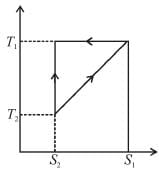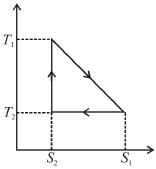The numerical values of the parameters T1 , T2 , S1 and S2 in the two figures are the same, then which cycle has greater efficiency?
Select one:

Detailed Solution for Second Law Of Thermodynamics MCQ Level – 2 (Part - 2) - Question 4

Efficiency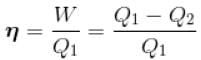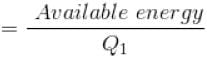For curve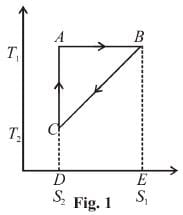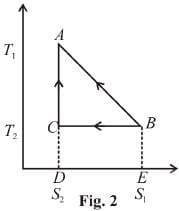Available energy per cycle is
Q1 – Q2 = Area of curve (ABC)
Q1 = Total area of curve (ABED)
⇒ Area of curve is same for both figures, but total area is more for Fig. 1.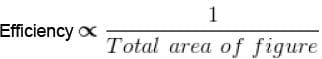Hence, second figure has greater efficiency.

Second Law Of Thermodynamics MCQ Level – 2 (Part - 2) - Question 5

An electric current of 3A flows through a resistance of 10 ohm. It is being cooled by running water and is kept at temperature 300K, change in entropy per second of the resistance :
Select one:

Detailed Solution for Second Law Of Thermodynamics MCQ Level – 2 (Part - 2) - Question 5

There is no change in the entropy of the resistance because there is no change in the state of wire.
The correct answer is: No change

Second Law Of Thermodynamics MCQ Level – 2 (Part - 2) - Question 6

Out of three Carnot engines, operating between reservoir temperature of (a) 400 and 500 K (b) 600 and 800 K (c) 400 and 600 K, which has the greater thermal efficiency?

Second Law Of Thermodynamics MCQ Level – 2 (Part - 2) - Question 7

A reversible engine cyclic is shown in the following T–S diagram. The efficiency of the engine is :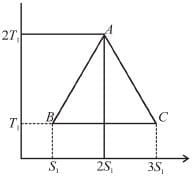Select one:

Detailed Solution for Second Law Of Thermodynamics MCQ Level – 2 (Part - 2) - Question 7

In given figure, available energy per cycle is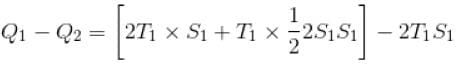= T1S1
and    Q1 = 3T1S1
efficiency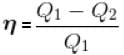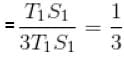Second Law Of Thermodynamics MCQ Level – 2 (Part - 2) - Question 8

An engine absorbs heat at temperature of 1000 K and rejects heat at 600 K. If the engine operates at maximum possible efficiency, the amount of work performed by the engine for 200 J heat input is
Select one:

Second Law Of Thermodynamics MCQ Level – 2 (Part - 2) - Question 9

An amount of heat Q is transferred from a heat reservoir at temperature TA to another heat reservoir at temperature TB. What is the change in the entropy Δs of the combined system?
Select one:

Detailed Solution for Second Law Of Thermodynamics MCQ Level – 2 (Part - 2) - Question 9

Conventionally, heat given to the system is taken to be positive and heat extracted from the system is taken to be negative.
When heat is changed in the system entropy changed by an amount,
∴ For A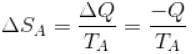Similarly for B,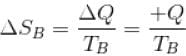ΔS = ΔSA + ΔSB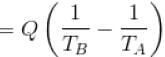The correct answer is: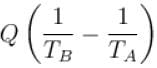Second Law Of Thermodynamics MCQ Level – 2 (Part - 2) - Question 10

The difference in entropy between a state of volume Vi and a state of volume Vf (temperature and number of molecules remaining constant) is equal to :
Select one:

## Topic wise Tests for IIT JAM Physics

217 tests
 Use Code STAYHOME200 and get INR 200 additional OFF Use Coupon Code
Information about Second Law Of Thermodynamics MCQ Level – 2 (Part - 2) Page
In this test you can find the Exam questions for Second Law Of Thermodynamics MCQ Level – 2 (Part - 2) solved & explained in the simplest way possible. Besides giving Questions and answers for Second Law Of Thermodynamics MCQ Level – 2 (Part - 2), EduRev gives you an ample number of Online tests for practice

217 tests